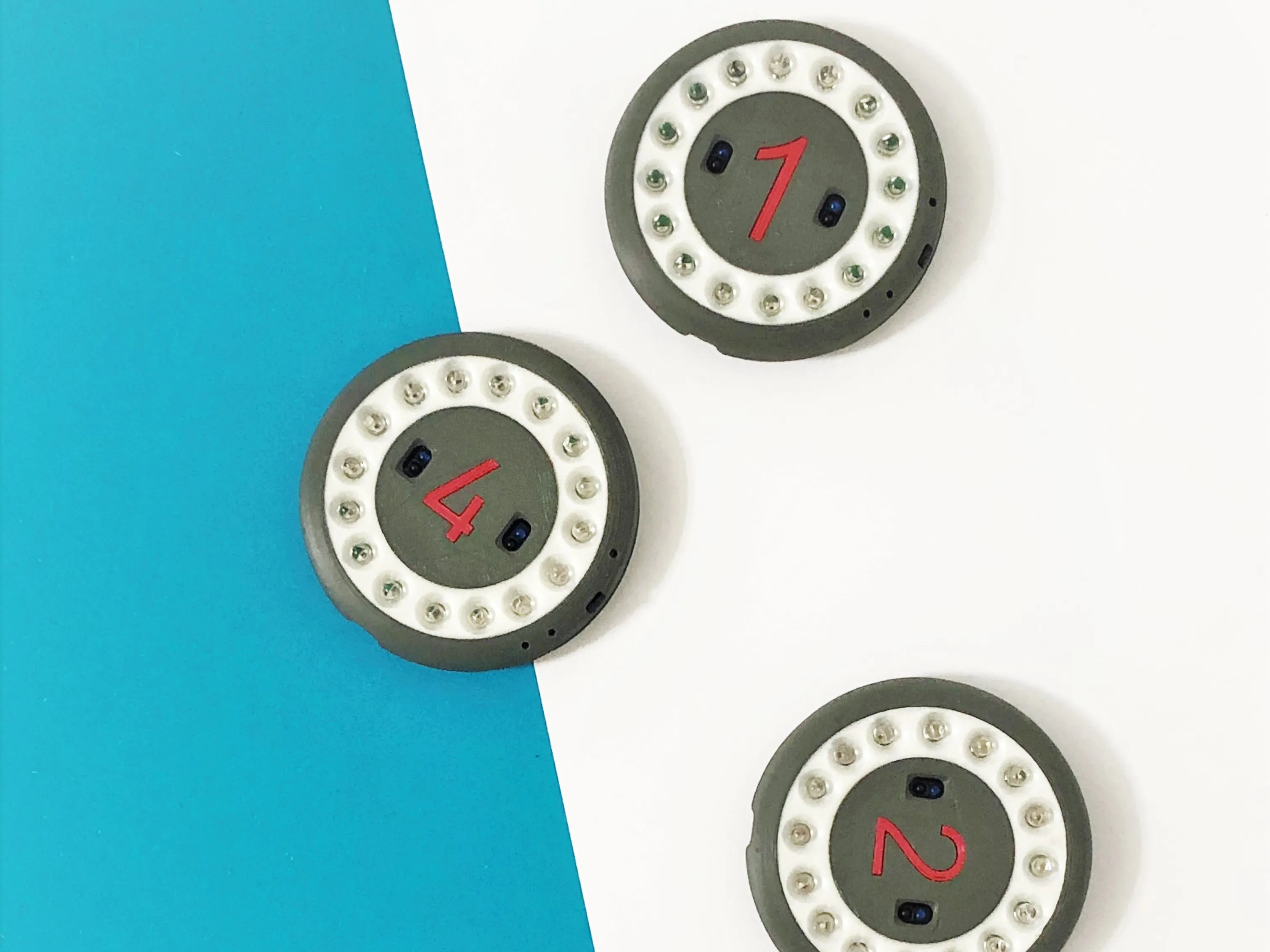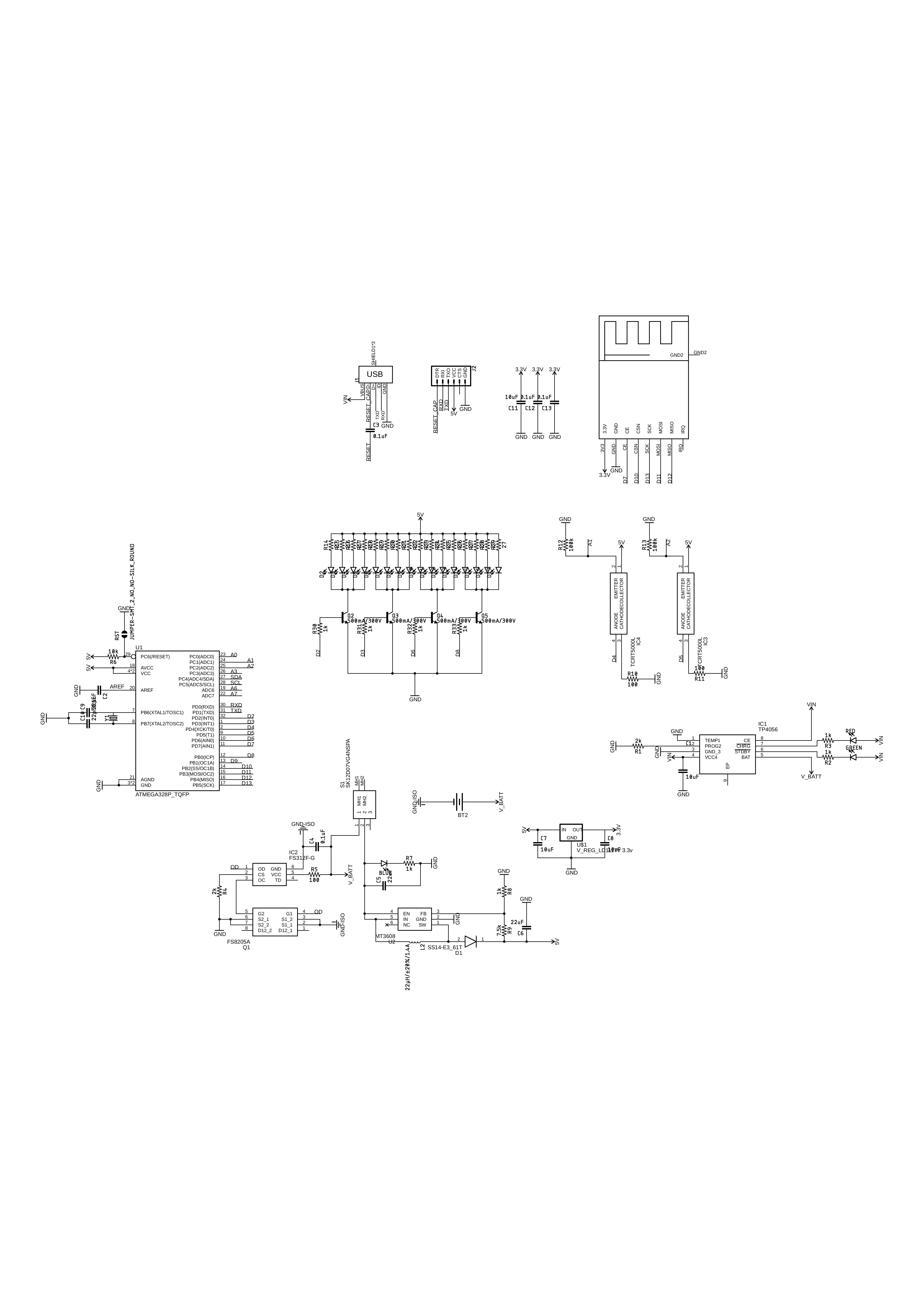Published © CC BY

# Reaction Training Dummy

As a request from an athlete friend to build cheap yet effective device to improve reaction training I came up with this!## Custom parts and enclosures

### Thingiverse

http://www.thingiverse.com/thing:3754409

## Schematics

### Eagle schematics## Code

### TransmitterNetwork_1.ino

Arduino
```#include <RF24Network.h>
#include <RF24.h>
#include <SPI.h>

// SENS stuff
int RawSensOn1;
int RawSensOn2;

int RawSensOff1;
int RawSensOff2;

int Sens1;
int Sens2;

// LEDS

byte LEDpins[] = {2,3,6,8};
int ledState = LOW;
int ledState2 = LOW;
unsigned long previousMillis = 0;        // will store last time LED was updated
long OnTime = 200;           // milliseconds of on-time
long OffTime = 200;          // milliseconds of off-time

boolean imON = true;

RF24 radio(7,10);                    // nRF24L01(+) radio attached using Getting Started board

RF24Network network(radio);          // Network uses that radio

const uint16_t this_node = 00;        // Address of our node in Octal format
const uint16_t node01 = 01;       // Address of the other node in Octal format
const uint16_t node011 = 011;       // Address of the other node in Octal format
const uint16_t node0111 = 0111;       // Address of the other node in Octal format

byte randNumber = this_node;

void setup(void)
{
//  Serial.begin(115200);

pinMode(4,OUTPUT);
pinMode(5,OUTPUT);

pinMode(A1, INPUT);
pinMode(A2, INPUT);

for (int x=0; x < 4; x++){
pinMode(LEDpins[x], OUTPUT);
}
randomSeed(analogRead(0)); //create unique seed value for random number generation

SPI.begin();
network.begin(/*channel*/ 90, /*node address*/ this_node);

powerUpLED();

//  Serial.println("Setup Done");
//  Serial.println("");
}

void loop() {

network.update();                          // Check the network regularly

//===== Receiving =====//
while ( network.available() ) { // Is there any incoming data?
byte incomingData;
//    Serial.print("Incoming - ");
//    Serial.println(incomingData);
if(incomingData == this_node){
imON = true;
randNumber = this_node;
}
}
if(imON == true){
unsigned long currentMillis = millis();

if((ledState == HIGH) && (currentMillis - previousMillis >= OnTime)){
ledState = LOW;  // Turn it off
ledState2 = HIGH;  // turn it on
previousMillis = currentMillis;  // Remember the time
digitalWrite(2, ledState);  // Update the actual LED
digitalWrite(6, ledState);  // Update the actual LED
digitalWrite(3, ledState2);  // Update the actual LED
digitalWrite(8, ledState2);  // Update the actual LED
}
else if ((ledState == LOW) && (currentMillis - previousMillis >= OffTime)){
ledState = HIGH;  // turn it on
ledState2 = LOW;  // Turn it off
previousMillis = currentMillis;   // Remember the time
digitalWrite(2, ledState);    // Update the actual LED
digitalWrite(6, ledState);  // Update the actual LED
digitalWrite(3, ledState2);  // Update the actual LED
digitalWrite(8, ledState2);  // Update the actual LED
}

digitalWrite(4,HIGH);     //turn On RECOGNITION LED
digitalWrite(5,HIGH);     //turn On RECOGNITION LED
delayMicroseconds(200);  //wait
digitalWrite(4,LOW);     //turn Off RECOGNITION LED
digitalWrite(5,LOW);     //turn Off RECOGNITION LED
delayMicroseconds(200);  //wait
RawSensOff1=analogRead(A1);        // again take reading from photodiode :noise
RawSensOff2=analogRead(A2);        // again take reading from photodiode :noise
Sens1=RawSensOn1-RawSensOff1;      //taking differnce:[ (noise+signal)-(noise)] just signal
Sens2=RawSensOn2-RawSensOff2;      //taking differnce:[ (noise+signal)-(noise)] just signal

if(Sens1 > 180 ||Sens2 > 180){
while(randNumber == this_node){
randNumber = random(0,4);
if(randNumber == 0) randNumber = this_node;
if(randNumber == 1) randNumber = node01;
if(randNumber == 2) randNumber = node011;
if(randNumber == 3) randNumber = node0111;
//         Serial.println(randNumber, OCT);
}
bool ok = network.write(header, &randNumber, 1); // Send the data
if (ok){
//        Serial.println("ok.");
imON = false;
powerDownLED();
}
else{
//        Serial.println("failed.");
}
}
}
}
void powerUpLED (){
for (int i = 0; i <= 4; i++) {
digitalWrite(2, HIGH);
delay(70);
digitalWrite(2, LOW);
delay(70);
digitalWrite(3, HIGH);
delay(70);
digitalWrite(3, LOW);
delay(70);
digitalWrite(6, HIGH);
delay(70);
digitalWrite(6, LOW);
delay(70);
digitalWrite(8, HIGH);
delay(70);
digitalWrite(8, LOW);
delay(70);
}
}
void powerDownLED (){

digitalWrite(2, LOW);
digitalWrite(3, LOW);
digitalWrite(6, LOW);
digitalWrite(8, LOW);
}
```

## Credits

### Marijo Blažević

2 projects • 17 followers
Electrical Hardware enthusiast
Thanks to Ino.